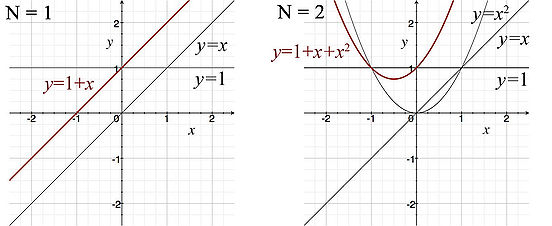# Science:Infinite Series Module/Units/Unit 3/3.1 Power Series/3.1.02 The Power Series

Indeed, a there is a more general representation for the series we introduced on the previous page:

$\sum _{k=1}^{\infty }ax^{k-1}=a+ax+ax^{2}+ax^{3}+\ldots ,\ a\neq 0$The more general representation is given by the following definition.

Definition: Power Series
An infinite series of the form

$\sum _{k=0}^{\infty }a_{k}(x-c)^{k},$where c is a constant, is a power series about c. The constants, ak are referred to as the coefficients of the series.

### What Is A Power Series?

It may help to consider a simple example with partial sums. Let's take the case where ak = 1, and c = 0, which gives us

$y(x)=\sum _{k=0}^{N}x^{k}$If we let N = 1, then we have a polynomial of order 1

$y(x)=\sum _{k=0}^{1}x^{k}=1+x$If we take N = 2, then we have a polynomial of order 2

$y(x)=\sum _{k=0}^{2}x^{k}=1+x+x^{2}$Similarly, with N = 3, then we would obtain a polynomial of order 3

$y(x)=\sum _{k=0}^{3}x^{k}=1+x+x^{2}+x^{3}$In the limit as N goes to infinity, we obtain a power series.

The figure below provides a graphical explanation of what a power series is: an infinite polynomial.This diagram helps to demonstrate the idea of a what a power series is. We consider the simple case when ak = 1, and c = 0. Using partial sums, the left corresponds to N = 1, and the right to N = 2. As we let N go to infinity, we obtain a power series.